# Measuring Planar Objects with a Calibrated Camera

This example shows how to measure the diameter of coins in world units using a single calibrated camera.

### Overview

This example shows how to calibrate a camera, and then use it to measure the size of planar objects, such as coins. An example application of this approach is measuring parts on a conveyor belt for quality control.

### Calibrate the Camera

Camera calibration is the process of estimating the parameters of the lens and the image sensor. These parameters are needed to measure objects captured by the camera. This example shows how to calibrate a camera programmatically. Alternatively, you can calibrate a camera using the Using the Single Camera Calibrator App app.

To calibrate the camera, we first need to take multiple images of a calibration pattern from different angles. A typical calibration pattern is an asymmetric checkerboard, where one side contains an even number of squares, both black and white, and the other contains an odd number of squares.

The pattern must be affixed to a flat surface, and it should be at approximately the same distance from the camera as the objects you want to measure. The size of a square must be measured in world units, for example millimeters, as precisely as possible. In this example we use 9 images of the pattern, but in practice it is recommended to use 10 to 20 images for accurate calibration.

### Prepare Calibration Images

Create a cell array of file names of calibration images.

```numImages = 9; files = cell(1, numImages); for i = 1:numImages files{i} = fullfile(matlabroot, 'toolbox', 'vision', 'visiondata', ... 'calibration', 'slr', sprintf('image%d.jpg', i)); end % Display one of the calibration images magnification = 25; I = imread(files{1}); figure; imshow(I, InitialMagnification = magnification); title("One of the Calibration Images");```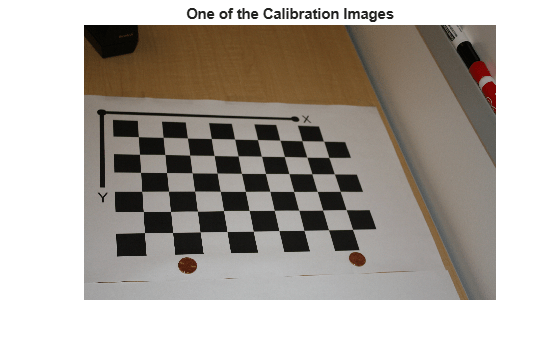### Estimate Camera Parameters

```% Detect the checkerboard corners in the images. [imagePoints, boardSize] = detectCheckerboardPoints(files); % Generate the world coordinates of the checkerboard corners in the % pattern-centric coordinate system, with the upper-left corner at (0,0). squareSize = 29; % in millimeters worldPoints = generateCheckerboardPoints(boardSize, squareSize); % Calibrate the camera. imageSize = [size(I, 1), size(I, 2)]; cameraParams = estimateCameraParameters(imagePoints, worldPoints, ... ImageSize = imageSize); % Evaluate calibration accuracy. figure; showReprojectionErrors(cameraParams); title("Reprojection Errors");```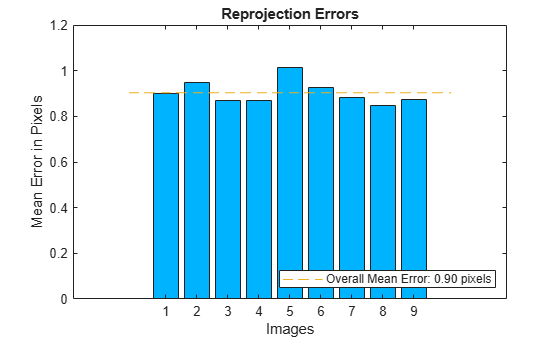The bar graph indicates the accuracy of the calibration. Each bar shows the mean reprojection error for the corresponding calibration image. The reprojection errors are the distances between the corner points detected in the image, and the corresponding ideal world points projected into the image.

### Read the Image of Objects to Be Measured

Load the image containing objects to be measured. This image includes the calibration pattern, and the pattern is in the same plane as the objects you want to measure. In this example, both the pattern and the coins are on the same table top.

Alternatively, you could use two separate images: one containing the pattern, and the other containing the objects to be measured. Again, the objects and the pattern must be in the same plane. Furthermore, images must be captured from exactly the same view point, meaning that the camera must be fixed in place.

```imOrig = imread(fullfile(matlabroot, "toolbox", "vision", "visiondata", ... "calibration", "slr", "image9.jpg")); figure; imshow(imOrig, InitialMagnification = magnification); title("Input Image");```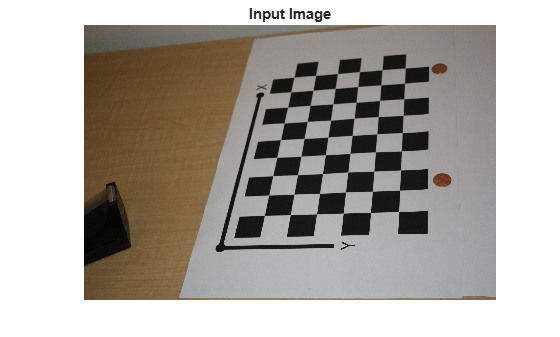### Undistort the Image

Use the cameraParameters object to remove lens distortion from the image. This is necessary for accurate measurement.

```% Since the lens introduced little distortion, use 'full' output view to illustrate that % the image was undistored. If we used the default 'same' option, it would be difficult % to notice any difference when compared to the original image. Notice the small black borders. [im, newOrigin] = undistortImage(imOrig, cameraParams, OutputView = "full"); figure; imshow(im, InitialMagnification = magnification); title("Undistorted Image");```Note that this image exhibits very little lens distortion. The undistortion step is far more important if you use a wide-angle lens, or a low-end webcam.

### Segment Coins

In this case, the coins are colorful on white background. Use the saturation component of the HSV representation of the image to segment them out.

```% Convert the image to the HSV color space. imHSV = rgb2hsv(im); % Get the saturation channel. saturation = imHSV(:, :, 2); % Threshold the image t = graythresh(saturation); imCoin = (saturation > t); figure; imshow(imCoin, InitialMagnification = magnification); title("Segmented Coins");```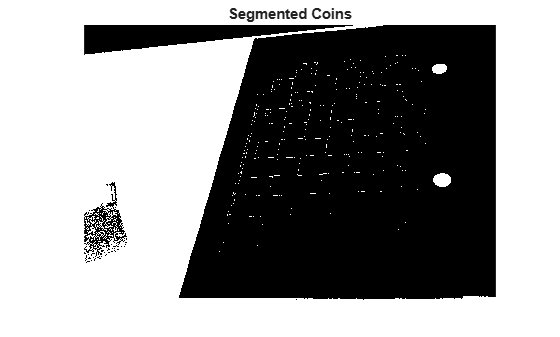### Detect Coins

We can assume that the two largest connected components in the segmented image correspond to the coins.

```% Find connected components. blobAnalysis = vision.BlobAnalysis(AreaOutputPort = true,... CentroidOutputPort = false,... BoundingBoxOutputPort = true,... MinimumBlobArea = 200, ExcludeBorderBlobs = true); [areas, boxes] = step(blobAnalysis, imCoin); % Sort connected components in descending order by area [~, idx] = sort(areas, "Descend"); % Get the two largest components. boxes = double(boxes(idx(1:2), :)); % Reduce the size of the image for display. scale = magnification / 100; imDetectedCoins = imresize(im, scale); % Insert labels for the coins. imDetectedCoins = insertObjectAnnotation(imDetectedCoins, "rectangle", ... scale * boxes, "penny"); figure; imshow(imDetectedCoins); title("Detected Coins");```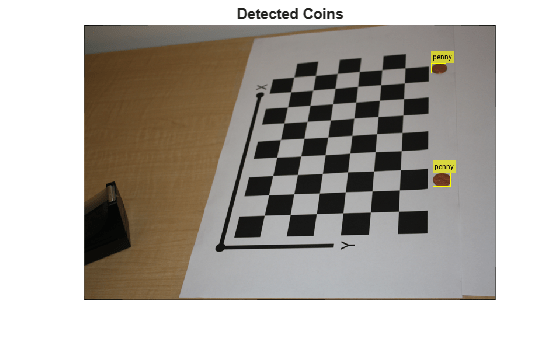### Compute Extrinsics

To map points in the image coordinates to points in the world coordinates we need to compute the rotation and the translation of the camera relative to the calibration pattern. Note that the `estimateExtrinsics` function assumes that there is no lens distortion. In this case imagePoints have been detected in an image that has already been undistorted using `undistortImage`.

```% Detect the checkerboard. [imagePoints, boardSize] = detectCheckerboardPoints(im); % Adjust the imagePoints so that they are expressed in the coordinate system % used in the original image, before it was undistorted. This adjustment % makes it compatible with the cameraParameters object computed for the original image. imagePoints = imagePoints + newOrigin; % adds newOrigin to every row of imagePoints % Extract camera intrinsics. camIntrinsics = cameraParams.Intrinsics; % Compute extrinsic parameters of the camera. camExtrinsics = estimateExtrinsics(imagePoints, worldPoints, camIntrinsics);```

### Measure the First Coin

To measure the first coin we convert the top-left and the top-right corners of the bounding box into world coordinates. Then we compute the Euclidean distance between them in millimeters. Note that the actual diameter of a US penny is 19.05 mm.

```% Adjust upper left corners of bounding boxes for coordinate system shift % caused by undistortImage with output view of 'full'. This would not be % needed if the output was 'same'. The adjustment makes the points compatible % with the cameraParameters of the original image. boxes = boxes + [newOrigin, 0, 0]; % zero padding is added for width and height % Get the top-left and the top-right corners. box1 = double(boxes(1, :)); imagePoints1 = [box1(1:2); ... box1(1) + box1(3), box1(2)]; % Get the world coordinates of the corners worldPoints1 = img2world2d(imagePoints1, camExtrinsics, camIntrinsics); % Compute the diameter of the coin in millimeters. d = worldPoints1(2, :) - worldPoints1(1, :); diameterInMillimeters = hypot(d(1), d(2)); fprintf("Measured diameter of one penny = %0.2f mm\n", diameterInMillimeters);```
```Measured diameter of one penny = 19.00 mm ```

### Measure the Second Coin

Measure the second coin the same way as the first coin.

```% Get the top-left and the top-right corners. box2 = double(boxes(2, :)); imagePoints2 = [box2(1:2); ... box2(1) + box2(3), box2(2)]; % Apply the inverse transformation from image to world worldPoints2 = img2world2d(imagePoints2, camExtrinsics, camIntrinsics); % Compute the diameter of the coin in millimeters. d = worldPoints2(2, :) - worldPoints2(1, :); diameterInMillimeters = hypot(d(1), d(2)); fprintf("Measured diameter of the other penny = %0.2f mm\n", diameterInMillimeters);```
```Measured diameter of the other penny = 18.85 mm ```

### Measure the Distance to The First Coin

In addition to measuring the size of the coin, we can also measure how far away it is from the camera.

```% Compute the center of the first coin in the image. center1_image = box1(1:2) + box1(3:4)/2; % Convert to world coordinates. center1_world = img2world2d(center1_image, camExtrinsics, camIntrinsics); % Remember to add the 0 z-coordinate. center1_world = [center1_world 0]; % Compute the distance to the camera. cameraPose = extr2pose(camExtrinsics); cameraLocation = cameraPose.Translation; distanceToCamera = norm(center1_world - cameraLocation); fprintf("Distance from the camera to the first penny = %0.2f mm\n", ... distanceToCamera);```
```Distance from the camera to the first penny = 719.52 mm ```

### Summary

This example showed how to use a calibrated camera to measure planar objects. Note that the measurements were accurate to within 0.2 mm.

### References

 Z. Zhang. A flexible new technique for camera calibration. IEEE Transactions on Pattern Analysis and Machine Intelligence, 22(11):1330-1334, 2000.